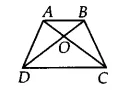Q

# Diagonals AC and BD of a quadrilateral ABCD intersect at O in such a way that ar (AOD) = ar (BOC). Prove that ABCD is a trapezium.

Q: 15    Diagonals AC and BD of a quadrilateral ABCD intersect at O in such a way that . Prove that ABCD is a trapezium.

ViewsWe have,
ABCD is a quadrilateral and diagonals AC and BD intersect at O such that ar(AOD) = ar (BOC) ...........(i)

Now, add ar (BOA) on both sides, we get

ar(AOD) + ar (BOA)  = ar (BOA) + ar (BOC)
ar (ABD) = ar (ABC)
Since the ABC and ABD lie on the same base AB and have an equal area.
Therefore, AB || CD

Hence ABCD is a trapezium.

Exams
Articles
Questions